# Dividing money

Jane and Silvia are to divide 1200 euros in a ratio of 19:11. How many euros does Jane have?

x =  320 Eur

### Step-by-step explanation: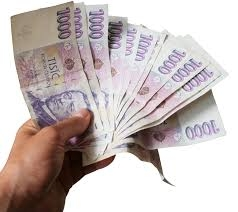Did you find an error or inaccuracy? Feel free to write us. Thank you!Tips to related online calculators
Check out our ratio calculator.

## Related math problems and questions:

• Divide moneyDivide 1200 USD at a ratio of 1:2:3:4:5:6:9:10
• Discount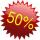Product has been discounted twice by 19%. What is the total discount given?
• Meanif the mean of the set of data 5, 17, 19, 14, 15, 17, 7, 11, 16, 19, 5, 5, 10, 8, 13, 14, 4, 2, 17, 11, x is -91.74, what is the value of x?
• Dividing moneyMilan divided 360 euros in the ratio 4: 5, Erik in the ratio 500: 625. How many euros did the individual parts of Milan and how many Erik?
• Division of moneyCalculate how many euros have Matthew, Miriam, Lucy, Michael, Janka when together have 2,700 euros and the amounts are at a ratio of 1:5:6:7:8.
• Suit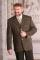The suit cost 119 euros. How much cost the pants if it is 50% cheaper than the jacket.
• ErikThree friends Peter, Erik and Milan shared 420 euros in a ratio of 1: 2: 3. How many euros did Erik get?
• Money split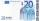Anton and Ferko got 2,500 euros together. The amount was split in a 1:4 ratio. How much did each get?
• EurosPeter, Jane, and Thomas have together € 550. Tomas has 20 euros more than Jane, Peter € 150 less than Thomas. Determine how much has each of them.
• Divide money 2Ben and Dan had the same amount of money at the start. When Ben gave 300 to Dan, the ratio of Ben's money to Dan's money became 2:3. How much money did each have at first?
• Jane and Miro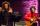Jane's brother Miro is 42 years. And he is three times old as it was Jane when Miro was for so many years as there are now Jane. How old is Jane?
• DivideDivide the number 72 in the ratio 7: 2 and calculate the ratio of the numbers found in this order and write down as decimal.
• Divide 5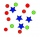Divide 288 in the following ratio 3 : 4 : 5
• RootThe root of the equation (x-19)2 -10 = x2 -11x is (equal or greater or less than zero)? ...
• Store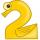Peter paid in store 3 euros more than half the amount that was on arrival to the store. When he leave shop he left 10 euros. How many euros he had upon arrival to the store?
• Markup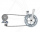A store pays \$370 for a bicycle.  The percent of markup is 19%.  What is the selling price of the bike?
• The price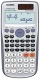The price of the calculator gradually decreased. From 65 euros to 30 euros, then to 10 euros and finally to 4 euros. What percentage were the individual reductions? What percentage of the original price was reduced by a total of?